Premium

# 2.NBT.A.3 Worksheets, Workbooks, Lesson Plans, and Games

#### CCSS.maths.CONTENT.2.NBT.A.3

:
"Read and write numbers to 1000 using base-ten numerals, number names, and expanded form."

Our Take:Second graders should be learning to represent numbers up to 1000 in a variety of different forms. For example, the student can represent the number 42 using place value terms (4 tens and 2 ones), expanded form (40 + 2), its number word (forty-two), and its standard form (42).

These worksheets and lesson plans can help students practise this Common Core State Standards skill.

## Worksheets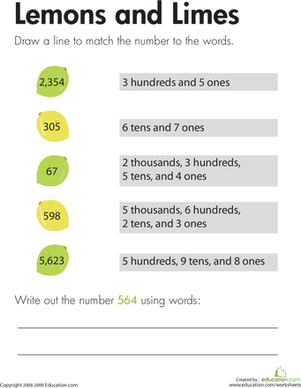Place Value: Lemons and Limes
Worksheet
Place Value: Lemons and Limes
Each sour fruit in this worksheet has a number written inside. Kids will match each number to the written equivalent of the number.
Year 3
Maths
Worksheet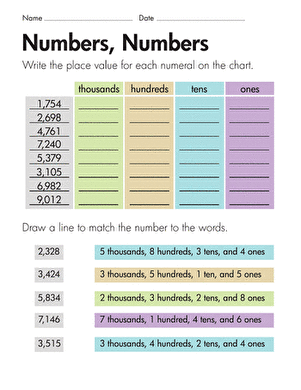Place Value: Numbers, Numbers
Worksheet
Place Value: Numbers, Numbers
For each four-digit number on this year three maths worksheet, kids determine the place value of each digit.
Year 3
Maths
Worksheet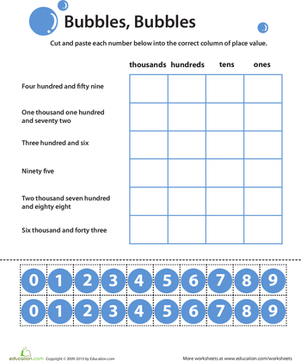Learning Place Value: Bubbles!
Worksheet
Learning Place Value: Bubbles!
In this year three maths worksheet, your child will learn about place value and practise reading and writing whole numbers up to the thousands.
Year 3
Maths
Worksheet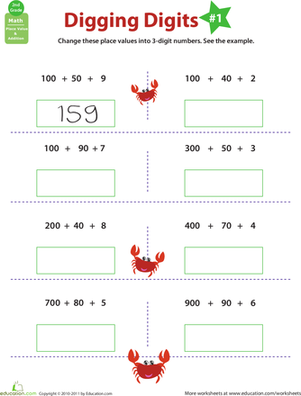Digging Digits: practise Place Value #1
Worksheet
Digging Digits: practise Place Value #1
Start your second grader out with some place value practise with this colorful worksheet that explores the ones, tens, and hundreds digits.
Year 3
Maths
Worksheet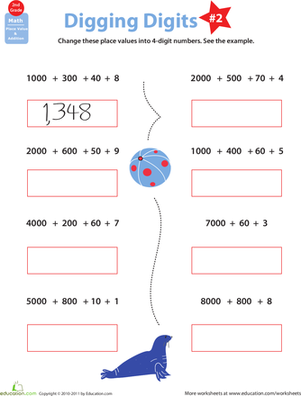Digging Digits: practise Place Value #2
Worksheet
Digging Digits: practise Place Value #2
Does your second grader need some help with place values? Check out this brightly-colored, fun worksheet that explores numbers up to four digits long.
Year 3
Maths
Worksheet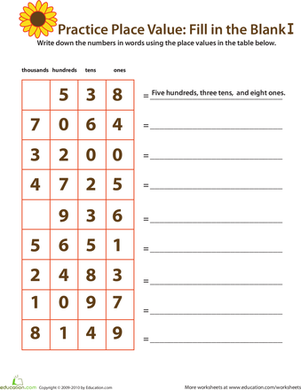Practise Place Value: Fill in the Blank I
Worksheet
Practise Place Value: Fill in the Blank I
Ready for a little place value fun? In this maths worksheet, your child will write out each number in words using place value notation.
Year 3
Maths
Worksheet

## Lesson Plans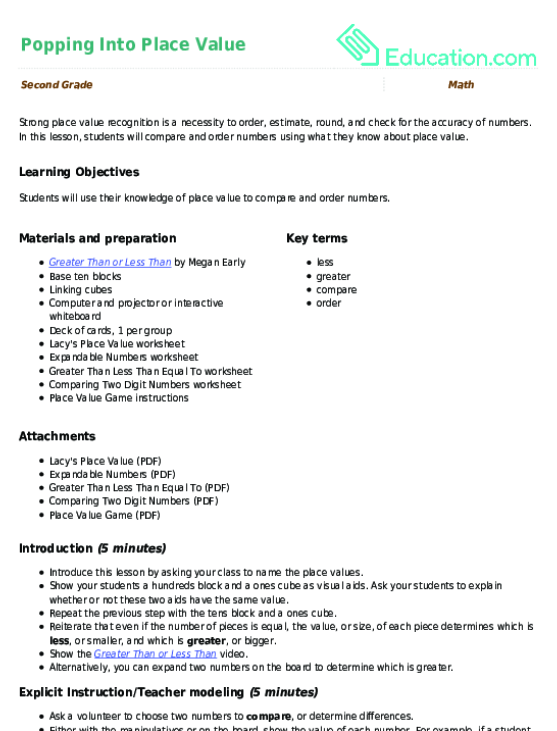Popping Into Place Value
Lesson plan
Popping Into Place Value
Strong place value recognition is a necessity to order, estimate, round, and check for the accuracy of numbers. In this lesson, students will compare and order numbers using what they know about place value.
Year 3
Maths
Lesson planBase-Ten Block Party
Lesson plan
Base-Ten Block Party
Help your students practise place value with this fun, hands-on base-ten block game. neighbourhood block teams compete to win points as they work together to show expanded numbers.
Year 3
Maths
Lesson plan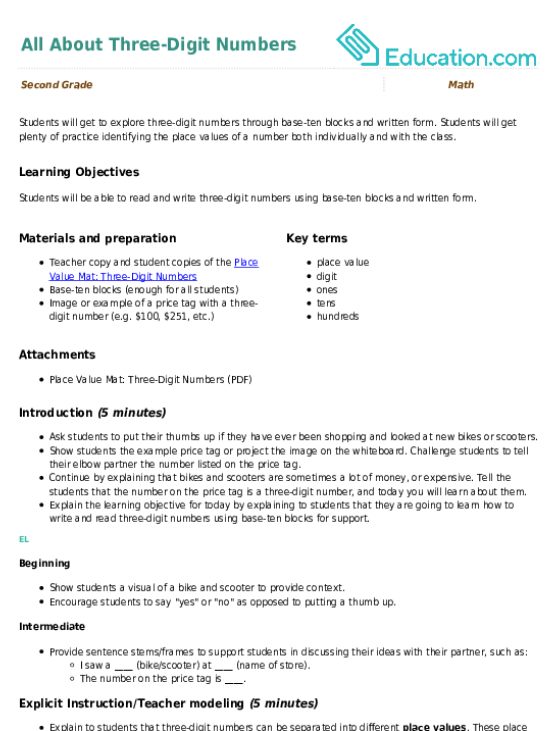All About Three-Digit Numbers
Lesson plan
All About Three-Digit Numbers
Students will get to explore three-digit numbers through base-ten blocks and written form. Students will get plenty of practise identifying the place values of a number both individually and with the class.
Year 3
Maths
Lesson planPlace Value Tower
Lesson plan
Place Value Tower
Students will learn how to find the actual value of three-digit numbers with this activity that has them use manipulatives to create the expanded form of a number.
Year 3
Maths
Lesson planNumerous Names for Numbers
Lesson plan
Numerous Names for Numbers
It is important for students to gain a better understanding of different ways to write numbers. In this lesson, your students will practise seeing numbers in various ways to help them comprehend the meaning of numbers.
Year 2
Maths
Lesson plan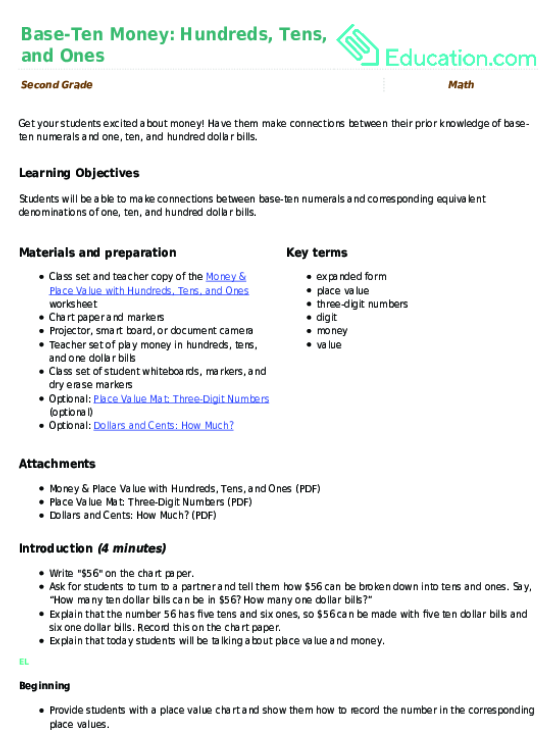Base-Ten Money: Hundreds, Tens, and Ones
Lesson plan
Base-Ten Money: Hundreds, Tens, and Ones
Get your students excited about money! Have them make connections between their prior knowledge of base-ten numerals and one, ten, and hundred dollar bills.
Year 3
Maths
Lesson plan

## Workbooks

No workbooks found for this common core node.

## Games

No games found for this common core node.

## Exercises

No exercises found for this common core node.

### Add to collection

Create new collection

0

### New Collection>

0Items

What could we do to improve Education.com?

Please note: Use the Contact Us link at the bottom of our website for account-specific questions or issues.

What would make you love Education.com?